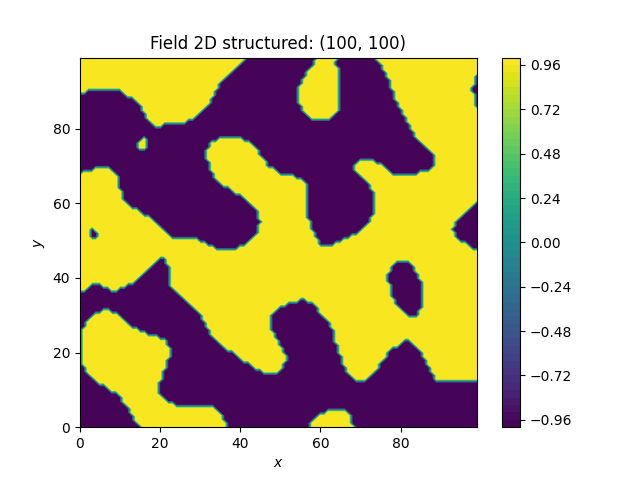# binary fields¶

Here we transform a field to a binary field with only two values. The dividing value is the mean by default and the upper and lower values are derived to preserve the variance.```import gstools as gs

# structured field with a size of 100x100 and a grid-size of 1x1
x = y = range(100)
model = gs.Gaussian(dim=2, var=1, len_scale=10)
srf = gs.SRF(model, seed=20170519)
srf.structured([x, y])
gs.transform.binary(srf)
srf.plot()
```

Total running time of the script: ( 0 minutes 1.080 seconds)

Gallery generated by Sphinx-Gallery